# A non-intrusive model order reduction approach for multi-physics parametrized problems - Application to induction heating process

Online since 02 April 2021

## Abstract

Finite element modeling (FEM) has recently become the most attractive computational tool to predict and optimize many industrial problems. However, the FEM becomes ineffective as far as complex multi-physics parameterized problems, such as induction heating process, are concerned because of high computational cost. This work aims at studying the possibility of applying a new approach based on the reduced order modeling (ROM) to obtain approximate solutions of a parametric problem. Basically, the effect of induction heating process parameters on some physical quantities of interest (QoI) will be analyzed under the real-time constraint. To achieve this dimensionality reduction, a set of precomputed solutions is first collected, at some sparse points in the space domain and for a properly selected process parameters, by solving the full-order models implemented in the commercial finite element software FORGE®. A Proper Orthogonal Decomposition (POD) based reducedorder model is then applied to the collected data to find a low dimensional space onto which the solution manifold could be projected and an approximated solution for new process parameters could be efficiently computed in real time. Besides, the POD is applied to build a reduced basis and to compute their corresponding modal coefficients. It is then followed by artificial intelligence techniques for regression purpose, such as sparse Proper Generalized Decomposition, to fit the low dimensional POD modal coefficients. Hence, the problem can be solved with a much lower dimension compared to the initial one. It was shown that a good approximation of the QoI was provided, in low-data limit, using a single POD modal coefficient as a response for the regression methods. However, the obtained approximation accuracy needs to be enhanced.

# 1 Introduction

Induction hardening is one of the most surface heat treatment processes widely employed in aerospace and automotive industries [1,2] to improve material performance by changing mechanical properties of the critical zones . The process consists of two steps; an electrically conducting component is first heated by electromagnetic induction to a temperature within or above the transformation range and then cooled by an immediate quenching. This process has the advantage of providing a very short surface heat-up times, a precise control of the treated zone, a good fatigue performance, and a good reproducibility . However, the control of mechanical parts quality goes through the prediction and the optimization of the induction hardening process. The main difficulty behind this optimization is the multi-physics property of induction hardening (electromagnetism, thermal, metallurgical, and mechanical field) in addition to the large number of process parameters; thanks to advanced numerical simulation tools, modelling and solving physical problems is possible by using some conventional discretization methods such as finite element, finite volume, etc. Nevertheless, passing through those methods to optimize multi-physics parametrized problems is often regarded as a key issue. In fact, when the number of parameters increases, the multi-query simulation approach becomes inefficient and makes optimization procedure very time consuming and computationally expensive. In order to alleviate such issues, reduced order modeling (ROM) techniques constitute an appealing alternative to standard discretization techniques given the interesting compromise in terms of computational cost, speed of execution, and results accuracy. Among these ROM techniques, which require in most cases the knowledge of equations describing the physics, Proper Orthogonal Decomposition (POD) , reduced basis methods , and Proper Generalized Decomposition (PGD)  have been widely used. However, some new strategies were developed to compute the parametric-based solution from sampled data, collected from experimental measurements or from finite element simulations, such as Sparse Subspace Learning (SSL)  and sparse Proper Generalized Decomposition (sPGD) .

The aim of this work is to investigate the possibility of applying a new approach based on the ROM to compute the temperature evolution in a gear under the effect of induction heating process parameters in almost real-time. To achieve this goal, the POD based reduced-order model was used and then followed by artificial intelligence (AI) techniques for regression purpose. It is worth pointing out that the proposed approach relies only on data and doesn’t require any knowledge of the full-order formulation or modification of the numerical finite element (FE) codes, hence the approximated parametric solution is constructed by using a datadriven non-intrusive ROM approach.

Based on a set of precomputed solutions of the full-order FE models (called snapshots), collected at some sparse points in the space domain and for different values of input parameters, the POD enables to build a reduced basis onto which the initial FE solution could be projected. The reduced state vector of the snapshots data, so-called POD modal coefficients, were then considered and multiple regression methods were used to fit the low dimensional POD modal coefficients. In other words, the POD modal coefficients represent the approximated solution of the ROM.

The rest of the paper is organized as follows: Section 2 defines the methodology and the numerical technologies with more details. Section 3 defines the process and the data generation. The results are then presented in Section 4 and Section 5 concludes this work.

# 2 Dimensionality reduction by POD and regression methods

Consider a set of P snapshots Ti = T(t, μi)i=1,…,P N, computed by solving the full-order FE model at each time step and for different values of input parameters µi, where t, µ = (µ1,…,µS), and N are the time, the set of S parameters, and the dimension of the FE solution, respectively. The snapshot matrix M NxP is defined such that M = [T1 T2 Tp] and each column contains a snapshot. To find the reduced basis, the singular value decomposition (SVD) is applied to M as follows:

where U ∈ ℝNxN and V ∈ ℝPxP are orthogonal matrices containing the left and right singular vectors of M, respectively. Σ ∈ ℝNxP is a rectangular diagonal matrix containing the singular values σk of M sorted in a decreasing order. The reduced POD basis, B = [ϕ1, ϕ2, …, ϕR], is defined as the first R left singular vectors of M (i.e. first R columns of U) corresponding to the R largest POD singular values. Thus, singular values provide a quantitative guidance for choosing the size of the POD basis. In practice, POD provides an efficient representation of the snapshot data in low-dimensional subspace of dimension R, much lower than N, such that

where ϕj and αji are called POD modes and POD modal coefficients, respectively. In matrix form, B ∈ ℝNxR and ai = BT.Ti where ai ∈ ℝR .

Now, instead of using P snapshots Ti, i=1,…,P of dimension N to fit the model to the data, the low-dimensional representation of the initial snapshots ai, i=1,…,P will be considered. Then, the return back to the original space could be achieved using Equation (2). The literature review illustrates that many regression techniques can be used to approximate the POD model coefficients for any choice of parameters included in the vector µ, such as sPGD which is based on the separated representation approach and enables quite rich approximations for high dimensional problems in a low-data limits , multiple linear regression , support vector regression SVR , random forests , and gradient boosting . These techniques will be applied in the current work.

Let now consider a database, composed by P combinations of input parameters µi and their corresponding response ai. The response matrix is written as A=[u1, …, uR] where each row of A contains a vector ai. Regression techniques consist in defining, in different ways, the approximated function fk as follows:

where εk is the residual term. The origin of regression error may depend on physical issues or the choice of hyperparameters associated to each method.

# 3 Problem statement and data generation

Induction heating (IH) is becoming one of the preferred heating technologies in many industrial applications  due to its advantages regarding fast heating, efficiency, accurate control, and cleanness compared to other classical heating techniques. Basically, it consists in applying an alternating current (AC) to a copper coil surrounding a conducting workpiece, a magnetic field generated by the AC induces an eddy current and consequently a heating by Joule effect of the workpiece (see Fig.1). In addition, IH combines multiple physics and it can be modeled by several FE codes, in which partial differential equations (PDE) describing electromagnetic and thermal problems are solved. Equations governing the physics at hand are not presented in this paper, more detailed information can be found in . In order to optimize and improve IH performances applied to a spur gear of 22 teeth (studied workpiece shown in Fig. 2), multiple parameters can be taken into consideration such as process, material, and geometrical parameters. However, as a first step of this study, three important parameters were considered while the other ones were kept constant. The selected parameters and their lower and upper limits are shown in Tables 1.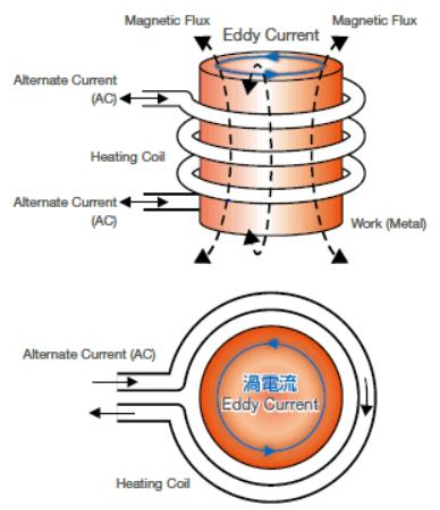Fig. 1. Principle of induction heating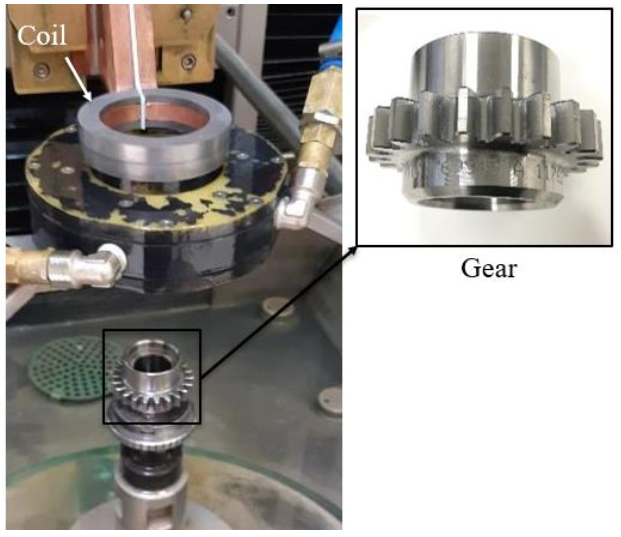Fig. 2. Experimental set-up of the induction heating.

Table 1. Input parameters and their lower and upper limits.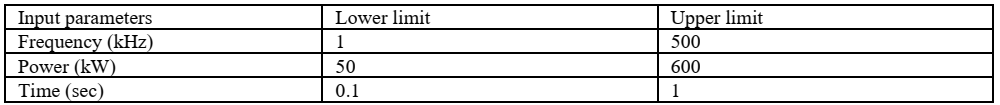As mentioned before, dimensionality reduction requires a set of precomputed high-fidelity solutions, collected by solving the original full-order model for different values of input parameters. For this reason, the commercial finite element software FORGE® was used. It is worth mentioning that the model has two symmetry planes and hence only half-tooth of gear is modeled for enhancing the computational efficiency. Besides, several FE simulations were conducted using Latin Hypercube Sampling (LHS) of experiments . Particularly, it guarantees a good coverage of each parameter space. According to the LHS, a total of 15 simulations have been generated. as shown in Fig. 3. Therefore, it is worth seeing how well the regression methods perform with small amount of data.

Fig. 3. Latin Hypercube Sampling

The post-processing of the temperature evolution was done at 14 specific points representing the main heat-affected zones as shown in Fig. 4.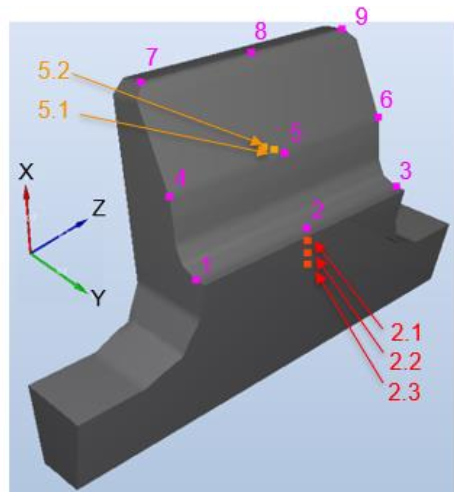Fig. 4. Measurement points.

# 4 Results and discussion

It is worth pointing out that the above-mentioned simulations result in different process times and consequently different dimensions. To alleviate such issue, a normalization of the discrete times was done for each simulation as follows: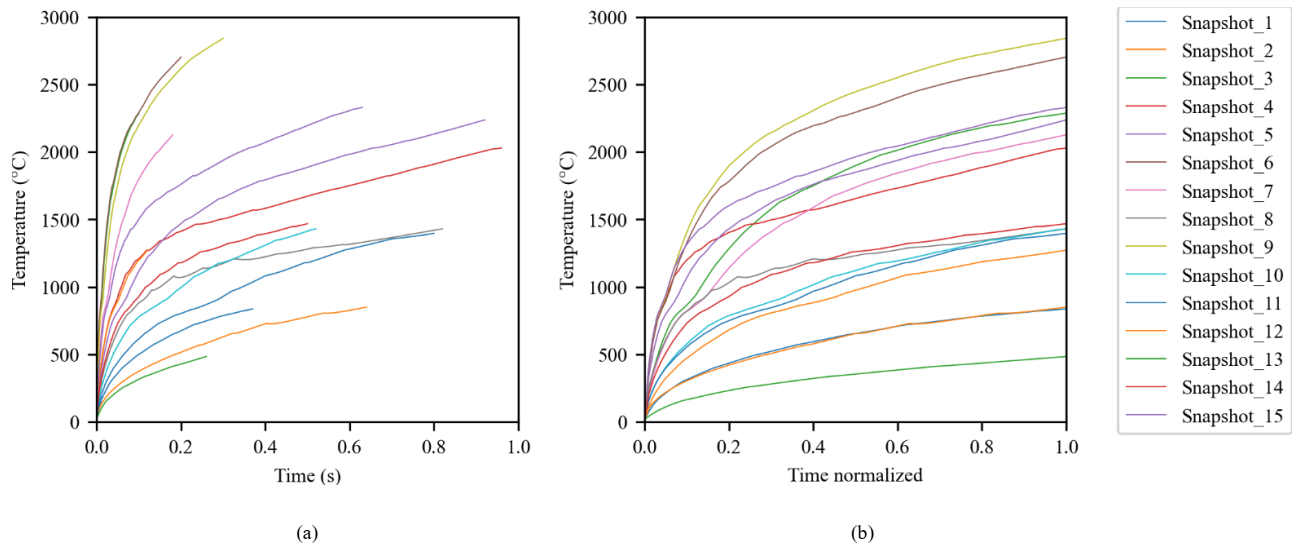Fig. 5. Temperature evolution at point #1 based on (a) the reel time, (b) the normalized time.

A snapshot matrix was defined for each measurement point, then the POD modes and their corresponding modal coefficients are computed as explained in Section 2. The left singular vectors of the snapshot matrices were truncated to the first singular vector which correspond to the POD mode. This choice is made in accordance with the fact that, with the first singular value, more than 90% of the variance is retained as shown in Fig. 6. It is worth pointing out that only results for points (#1, #5, #9, and #2.3) were shown in this section for the sake of clarity.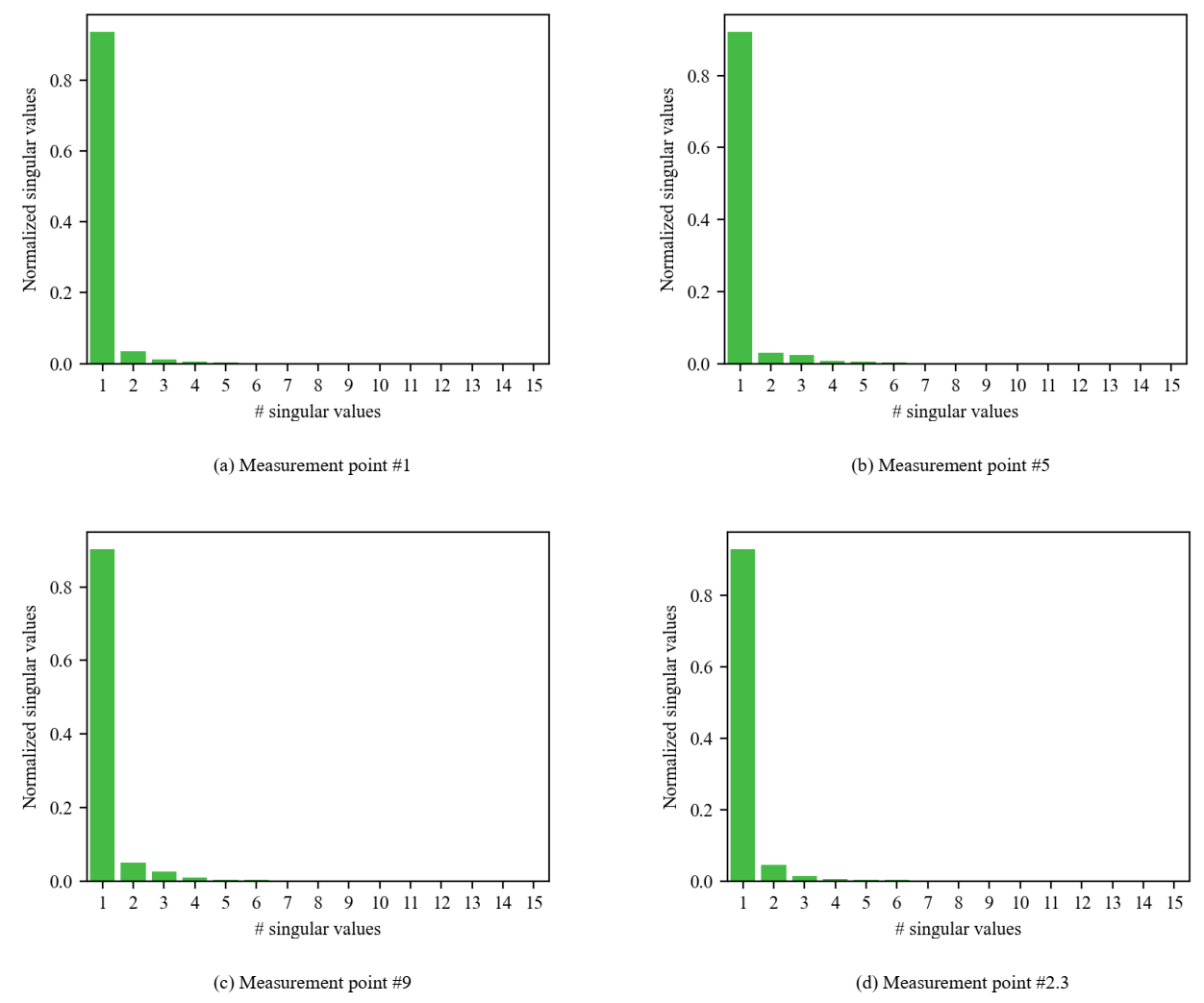Fig. 6. Normalized singular values of the thermal field for 4 snapshot matrices.

After computing the modal coefficients, a surrogate model for each one of them and for each measurement point was constructed. Before that, the standardization of the input parameters was applied to avoid problems related to units and different scaled features, then the dataset was divided into training and testing subsets (80% of data is used to build the models and 20% to evaluate their accuracy).

Fig. 7. shows the scaled real versus the scaled predicted values of modal coefficients for points (#1, #5, #9, and #2.3) using different regression methods implemented in python packages except the sPGD. The red points correspond to the data used to build the regression model and the blue ones correspond to the testing data used to evaluate its accuracy. When points are too close to the black line, the surrogate model provides a good fit to data. Indeed, the dispersion of these points with respect to the black line gives a visual indicator of error. Additionally, the score associated to each regression model for the red and blue points was also presented in Fig.7. It is clear that the results are slightly different from one measurement point to another and the SVR regression model provides the best fit followed by the sPGD, then the other techniques.

Fig. 7. Scaled predicted versus scaled real response for 4 measurement points.

Fig. 8. shows the temporal evolution of the temperature obtained by the full model, the POD-based reduced model (i.e. Using equation (2) with real values of modal coefficients), and the SVR regression model (i.e. using equation (2) with the predicted values of model coefficients) which provides comparatively the best fit.

It is worth mentioning that the curves of the SVR regression model (red curves) should overlap the dashed black curves which is almost perfectly done for both the training (left data) and testing data (right data) and for the four measurement points as well. For point #2.3, a slight difference between the red and black dashed curves of the test data was obtained. However, this error is still acceptable, and the predicted and the real curves show the same trend. Nevertheless, since one POD mode and consequently one model coefficient as a response for the regression was considered, it seems that it is not sufficient to describe in a proper way the real temporal evolution of temperature. To improve the approximation accuracy, two modes and modal coefficients were considered instead of one, but the obtained results of regression were not good enough and leading to a problem of overfitting, despite the optimization of the hyperparameters associated to regression techniques. This part constitutes a work in progress.

Fig. 8. Comparison of the temporal evolution of temperature between the initial full model, the POD-based reduced model (with the real values of model coefficients), and the SVR regression model (with the predicted values of model coefficients) for 4 measurement points: data used for creating the regression model (left) and data used to evaluate the regression model accuracy (right).

# 5 Conclusion

In this paper, an approach, based on dimensionality reduction by POD coupled with regression techniques to fit a model to the POD modal coefficients, was proposed to compute the temperature evolution during the multi-physics parametric-based induction heating process in low-data limit. The approach was successfully applied for 14 sparse measurement points in the space domain, in which a basis with a single vector was built and consequently a single POD modal coefficient was computed and used for creating the surrogate model. A good approximation was provided, and a quite good performance of some regression techniques was shown as well. A comparative investigation showed that the SVR regression model gives the best fit to the data.

Nevertheless, regression methods work well with the first POD modal coefficient associated to the first POD mode, but this latter doesn’t fit perfectly the initial FE solution. Applying regression methods to the second and third modal coefficients do not provide good results. Future developments are required in order to improve the accuracy of the approximated solution. Once a better approximation is obtained, the solution will be extended to address the whole space domain using interpolation techniques.

# Acknowledgements

This work was conducted with the help of the French Technological Research Institute for Materials, Metallurgy and Processes (IRT M2P). The authors would like to acknowledge IRT- M2P and the partners of the project TRANSFUGE led by IRT M2P.

 Krause, C., and Biasutti, F. (2014). Induction Hardening for the Aeronautic and Aerospace Industry. ASM HANDBOOK. vol. 4C.

DOI:https://doi.org/10.31399/asm.hb.v04c.9781627081672

 Rokicki, P. (2017). Induction hardening of tool steel for heavily loaded aircraft engine components. Arch. Metall. Mater. 62, 1, 315-320. DOI: 10.1515/amm-2017-0047

 Rudnev, V., Loveless, D., Cook, R. and Black, M. (2003). Handbook of Induction Heating. Marcell Dekker Inc., New York.

 Spezzapria, M. Multiphysical Finite Element Simulation of Contour Induction Hardening of Gears. PhD thesis, UNIVERSITÀ DEGLI STUDI DI PADOVA, 2016.

 Astrid, P. Reduction of Process Simulation Models: a proper orthogonal decomposition approach. PhD thesis, Eindhoven: Technische Universiteit Eindhoven. https://doi.org/10.6100/IR581728, 2004.

 Chinesta, F., Huerta, A., Rozza, G., and Willcox, K. (2017). Model reduction methods. Encyclopedia of Computational Mechanics Second Edition. p. 1–30. DOI:10.1002/9781119176817.ecm2110.

 Chinesta, F., Keunings, R., and Leygue, A. (2013). The proper generalized decomposition for advanced numerical simulations a primer. Springerbriefs. SpringerCham: Heidelberg, New York, Dordrecht.

 Borzacchiello, D., Aguado, J. V., and Chinesta, F. (2017). Non-intrusive Sparse Subspace Learning for Parametrized Problems, Archives of Computational Methods in Engineering: State-of-the-Art Reviews, pp. 1–24.

 Ibanez, R., Abisset-Chavanne, E., Ammar, A., Gonzalez, D., Cueto, E., Huerta, A., Duval, J L., and Chinesta, F. (2018). A multi-dimensional data-driven sparse identification technique : the sparse proper generalized decomposition. Hindawi Complexity 2018 :5608286, p. 1–11.

 Limousin, V., Delgerie, X., Leroy, E., Ibanez, R., Argerich, C., Daim, F., Duval, J L., and Chinesta, F. (2019). Advanced model order reduction and artifcial intelligence techniques empowering advanced structural mechanics simulations: application to crash test analyses. technical report. p. 1–33.

 Gülden Kaya Uyanık and Neşe Güler. (2013). A Study on Multiple Linear Regression Analysis. Procedia - Social and Behavioral Sciences 106:234–240.

 Awad, M. and Khanna, R. (2015). Support Vector Regression. In: Efficient Learning Machines. Apress, Berkeley, CA. https://doi.org/10.1007/978-1-4302-5990-9_4

 Cutler, A. Cutler, D R. and Stevens, J R. (2011). Random Forests. In: Zhang, C. and Ma, Y. (Ed.). Ensemble Machine Learning: Methods and Applications (pp.157-176).

 Natekin, A. and Knoll, A. (2013). Gradient boosting machines, a tutorial. Front. Neurorobot. 7:21. doi: 10.3389/fnbot.2013.00021.

 Lucia, Oscar and Maussion, Pascal and Dede, Enrique and Burdio, Jose M. (2013). Induction Heating Technology and Its Applications: Past Developments, Current Technology, and Future Challenges. IEEE Transactions on Industrial Electronics, vol. 61 (n°5). pp. 2509-2520.

 Cardinaux, D. Etude et modélisation numérique 3D par éléments finis d’un procédé de traitement thermique de toiles embouties après chauffage par induction: Application à un renfort de pied central automobile. PhD thesis, Mines ParisTech, 2008.

 Nippon Avionics Co.,Ltd, Principle of High-frequency Induction Heater Unit, sur https://www.avio.co.jp/english/products/ assem/ principle/ highfrequency/index.html, consulted on 04/12/2020 at 2pm.

 Stein, M. Large sample properties of simulations using latin hypercube sampling. Department of Statistics University of Chicago TECHNOMETRICS, vol. 29,NO. 2, 1987.

### Electronic reference

Khouloud Derouiche, Francisco Chinesta, Monzer Daoud and Khalil Traidi, « A non-intrusive model order reduction approach for multi-physics parametrized problems - Application to induction heating process », ESAFORM 2021 [Online], Online since 02 April 2021, connection on 28 November 2023. URL : https://popups.uliege.be/esaform21/index.php?id=1572

Khouloud Derouiche

Arts et Metiers Institute, 151 Boulevard de l'Hôpital, 75013 Paris, France. French Technological Research Institute for Materials, Metallurgy and Processes (IRT- M2P), 4 rue Augustin Fresnel, 57070 Metz.

Corresponding author: Khouloud.derouiche@ensam.eu

Francisco Chinesta

Arts et Metiers Institute, 151 Boulevard de l'Hôpital, 75013 Paris, France.

#### By this author

• Published in ESAFORM 2021

• Published in ESAFORM 2021

• Published in ESAFORM 2021

Monzer Daoud

French Technological Research Institute for Materials, Metallurgy and Processes (IRT- M2P), 4 rue Augustin Fresnel, 57070 Metz, France.

Khalil Traidi

Safran Tech, Rue des jeunes Bois, 78117 Châteaufort, France.

## Details

Title
A non-intrusive model order reduction approach for multi-physics parametrized problems - Application to induction heating process
Language
en
Author, co-author
Khouloud Derouiche, Francisco Chinesta, Monzer Daoud and Khalil Traidi,
Publication date
02 April 2021
Journal title
ESAFORM 2021
CC-BY
DOI
10.25518/esaform21.1572
Permanent URL
https://popups.uliege.be/esaform21/index.php?id=1572

## Statistics

Views : 875 (1 ULiège)